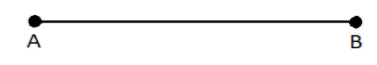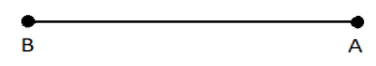QuestionAnswers

# Fill in the blank:Segment AB is ____________ segment BA.Verified
128.7k+ views
Hint: A line segment is bound between two fixed points A and B such that the line can be expressed as AB or BA without any change in its length.

In this question we need to establish the relation of a line segment AB with its reverse line segment BA. Line segment, as we know, is bound between two fixed points. In this case, the line segment is bound between the fixed points A and B as follows:So, the line segment AB starts from point A and ends at point B.
Let the length of distance between the points A and B be $6cm$ , then it means that the length of $AB = 6cm.$. Now, if we interchange the fixed points A and B and re draw the line then it will become:So now the line segment will become BA which starts from point B and ends at point A.
We have just interchanged the fixed points and not the length of the line. So BA is bound between the same points B and A without any change in the length of the distance between these two points.
That means $AB = 6cm = BA$ , so BA is also 6cm.
Hence line segment AB is the same as line segment BA as it passes through the same two points A and B.
Therefore we can conclude that the length of the line segment bound between two points stays the same, even if the points are reversed. Hence, segment AB is equal to segment BA.
Thus, we will use the word equal to fill in the blank.

Note: A line and line segment are not the same. While a line segment is bound between two fixed points. A line has no such fixed point, that is it can go up to infinity on either side. So, its length is infinite. The length of a line segment is fixed , whereas the length of a line cannot be measured because it has no definite length. Hence the length of a line segment is finite as it is fixed, whereas the length of a line is infinite as it can never be fixed.